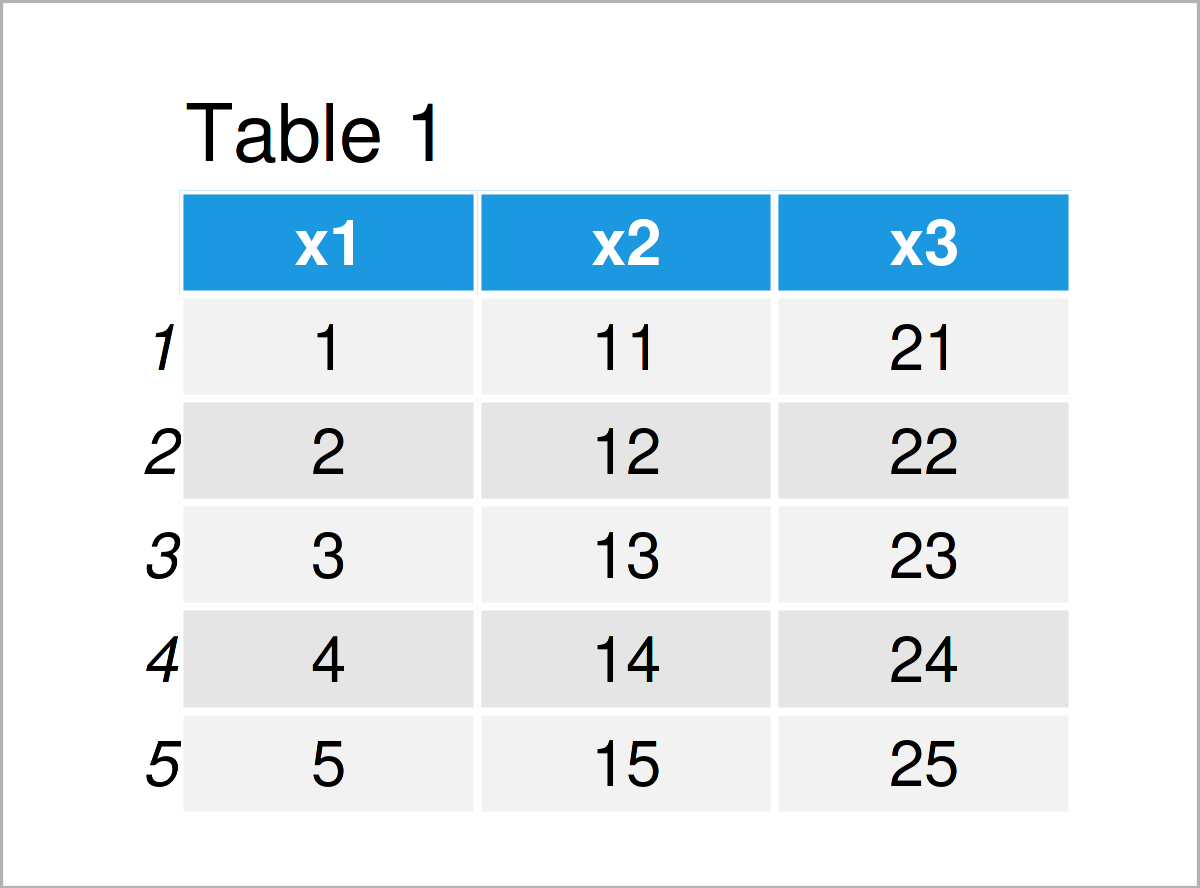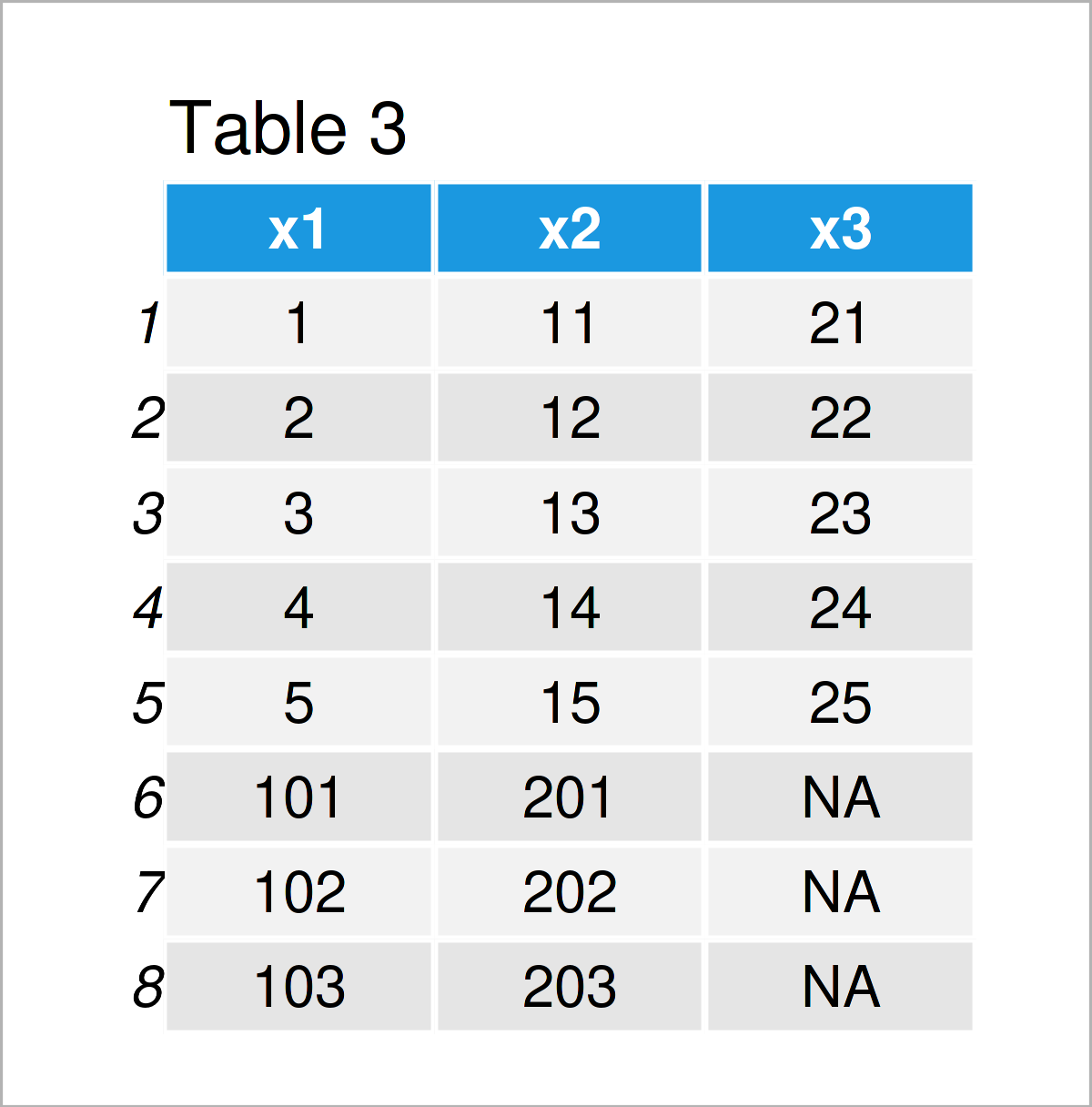# R Error in rbind(deparse.level, …) : numbers of columns of arguments do not match (2 Examples)

In this R tutorial you’ll learn how to fix the “Error in rbind(deparse.level, …) : numbers of columns of arguments do not match”.

The tutorial looks as follows:

Let’s dive right in…

## Example Data

We’ll use the following data frames as a basis for this R programming tutorial:

```data1 <- data.frame(x1 = 1:5,                # Create first example data
x2 = 11:15,
x3 = 21:25)
data1                                        # Print first example data``````data2 <- data.frame(x1 = 101:103,            # Create second example data
x2 = 201:203)
data2                                        # Print second example data```In Tables 1 and 2 you can see that we have created two data frames containing different values and partly overlapping column names.

## Example 1: Reproduce the Error in rbind – numbers of columns of arguments do not match

In this example, I’ll show how to replicate the “Error in rbind(deparse.level, …) : numbers of columns of arguments do not match” in the R programming language.

Let’s assume that we want to row-bind our two example data frames using the rbind function as shown below:

```data_rbind <- rbind(data1, data2)            # Try to combine data sets
# Error in rbind(deparse.level, ...) :
#   numbers of columns of arguments do not match```

As you can see, the previous R code has returned the error message “numbers of columns of arguments do not match”.

The reason for this error is that our two input data sets contain different columns. The first data frame contains three columns called x1, x2, and x3, and the second data frame contains only the columns x1 and x2.

Next, I’ll show a simple solution for this problem – so keep on reading!

## Example 2: Solve the Error in rbind – numbers of columns of arguments do not match

In Example 2, I’ll illustrate how to debug the “Error in rbind(deparse.level, …) : numbers of columns of arguments do not match”.

First, we need to install and load the dplyr package:

```install.packages("dplyr")                    # Install & load dplyr package
library("dplyr")```

Now, we can apply the bind_rows function of the dplyr package to stack our two data frames on top of each other:

```data_bind_rows <- bind_rows(data1, data2)    # Apply bind_rows function
data_bind_rows                               # Print stacked data frames```The output of the previously shown code is shown in Table 3: As you can see, we have managed to create a combined version of our two input data frames. The column x3 that is only contained in the first data frame has been filled up with NA values for the rows corresponding to the second data frame.

## Video, Further Resources & Summary

In case you need more information on the R code of this article, you may want to have a look at the following video on my YouTube channel. In the video tutorial, I demonstrate the R programming code of this post in RStudio:

In addition, you might want to read the related articles on this website. You can find some interesting tutorials that are related to the dealing with the “Error in rbind(deparse.level, …) : numbers of columns of arguments do not match” below.

Summary: In this R tutorial you have learned how to debug the “Error in rbind(deparse.level, …) : numbers of columns of arguments do not match”. Tell me about it in the comments section, in case you have any additional questions. Furthermore, don’t forget to subscribe to my email newsletter in order to get updates on the newest articles.

Subscribe to the Statistics Globe Newsletter